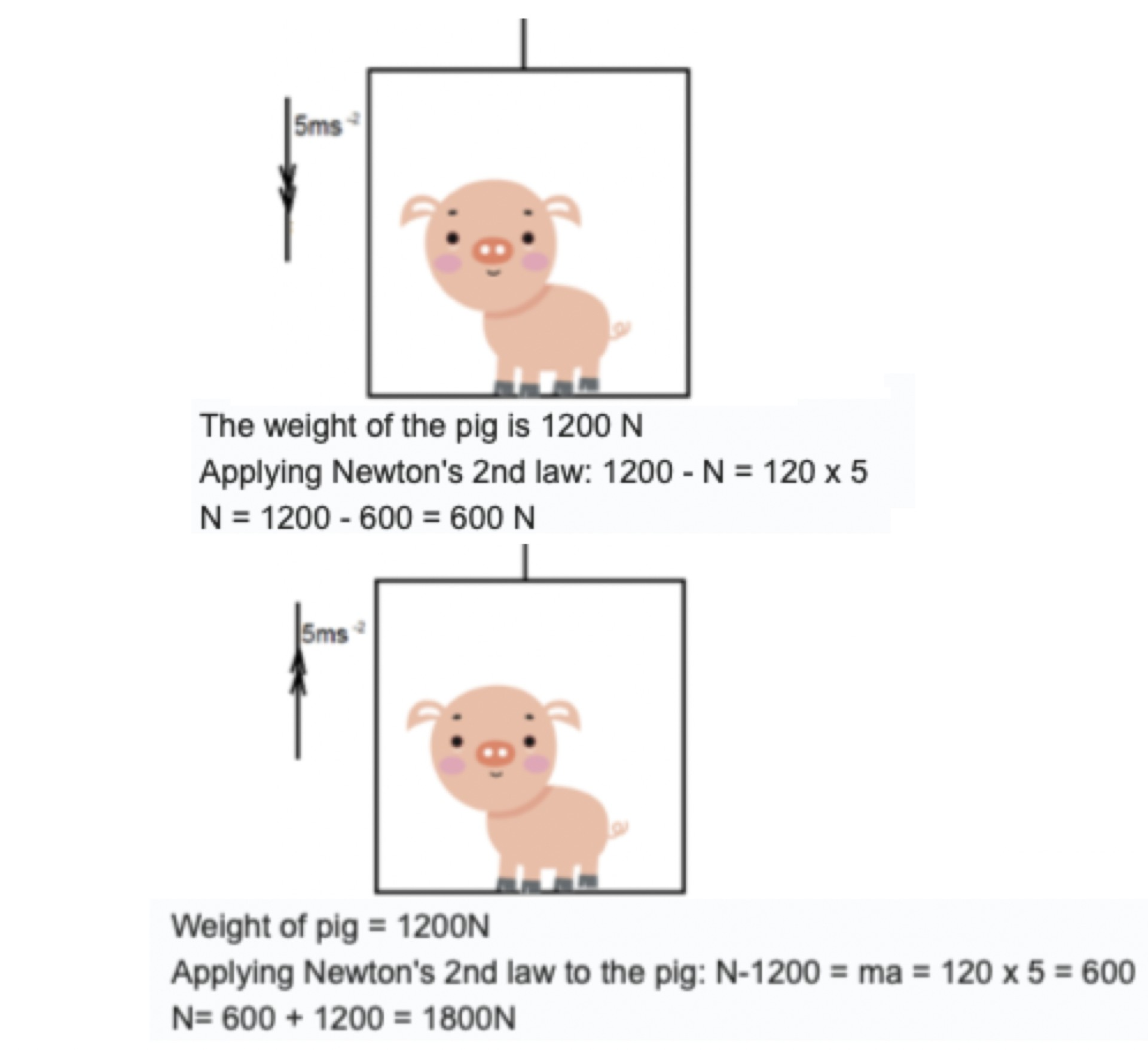# Newton´s pigsOne of my student's sent me a question about the classic pig in an elevator problem."Hi Chris, how can it be that the normal force is calculated these two different ways when the elevator is accelerating downwards and upwards?"

the weight of the pig is constant, mg
the resultant = ma (newt.2)
if up is positive in 1. N + -mg = ma so N = ma + mg
2. N + -mg = -ma so N = mg - ma

I find it easier to take the direction of accel. as positive
1. accel up implies N is bigger than mg so N - mg = ma so N = ma + mg
2. accel. down implies that mg is bigger than N so mg - N = ma so N = mg - ma

Really the answer to your question is because Newton's 2nd law says so, don't overthink it. You may feel that this is wrong but it's your intuition that is wrong not Newton. The Normal force is calculated in the same way but the result is different. The normal force is not constant; it depends how hard you press the surfaces together. When the elevator accelerates up the floor is pushing the pig with a force bigger than mg. When the elevator accelerates down, the floor pushes with a force less than mg.
Imagine you are lifting a box, the force you exert on the box is the normal force, think how you can make that bigger or smaller than the weight and what would happen to the box.

I think this is a good example of how a students' intuition sometimes gets in the way of understanding physics. When the pig is at rest the Normal force equals the weight. I think the student took this to mean that they are always equal, this caused confusion when trying to understand how these two forces can add to give a different resultant. The moral to the story is:

Don't think just apply the law.

v